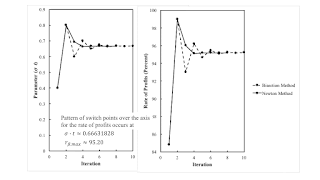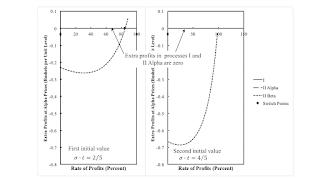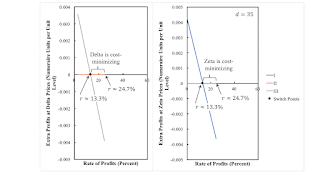## Saturday, August 28, 2021

### How To Find Fluke Switch PointsFigure 1: Convergence to a Pattern of Switch Points over the Axis for the Rate of Profits
1.0 Introduction

This post illustrates how to find fluke switch points. As usual, I proceed by example, in this case, as taken from my paper in Structural Change and Economic Dynamics.

2.0 Technoplogy

In this example of a capitalist economy, two commodities, iron and corn, are produced. One process is known for producing iron. In the iron industry, workers use inputs of iron and corn to produce an output of iron. The output of the iron industry is one ton with the inputs shown in Table 1. Two processes are known for producing corn. Each corn-producing process shown in Table 1 produces an output of one bushel corn from inputs of labor power, iron, and corn. Assume constant returns to scale.

 Input Industry Iron Corn I II Alpha II Beta Labor a0,1 = 1 a0,2α = (5191/5770) e1/10 - σt a0,2β = 305/494 Iron a1,1 = 9/20 a1,2α = (1/40) e1/10 - σt a1,2β = 3/1976 Corn a2,1 = 2 a2,2α = (1/10) e1/10 - σt a2,2β = 229/494

3.0 Switch Points

I take corn as the numeraire. Wages are paid out of the surplus at the end of the harvest. I take the rate of profits as given. In this post, I do not explain how to find the price of iron and the wage for, say, the Alpha technique, given the technology at a given value of (σ t).

At a switch point, no excess profits or costs arise in evaluating the Beta process in the corn industry at Alpha prices. That is, switch points are the roots of the following equation.

1 - {[p1αt) a1,2β + a2,2β](1 + r) + wαt) a0,2β} = 0

The above is a quadratic equation for this example. Let fkt) denote the kth root of the above equation.

rk = fkt)

These are the switch points for a specific value of the parameters σ t. I fix σ at 1/10. Figure 2 graphs the switch points, as well as the maxmimum wage, against time. You can see technical progress brings about reswitching and takes it away.Figure 2: Fluke Switch Points Partition Time

4.0 Numerical Methods

Various fluke cases arise in the example. They can be found by numerical methods. Table 2 defines, for four types of fluke cases, a new function whose root is the parameter values that correspond to the type of fluke case. For illustration, consider the fluke switch point that arises on the axis for the rate of profits with σ t ≈ 0.6663189. That is, gt) is the difference between the maximum rate of profits for the Beta technique and the rate of profits for a selected switch point for the Alpha and Beta techniques.

 Function Fluke Case gk(σ t) = fk(σ t) + 1 Pattern of switch points for the reverse substitution of labor. gk(σ t) = fk(σ t) Pattern of Switch points over the wage axis. gk(σ t) = rmax, β - fk(σ t) Pattern of switch points over the axis for the rate of profits. gk(σ t) is the discriminant of the quadratic equation above Reswitching pattern of switch points.

One needs two initial parameter values to start either algorithm specified here. Figure 3 graphs extra profits in operating the corn process in the Beta technique, as evaluated at Alpha prices. Extra profits are shown for two different parameter values. On the left panel, a switch point exists for a rate of profits smaller than the maximum rate of profits. The parameter values are evidently too small for a pattern of switch points over the axis for the rate of profits. On the right panel, the parameter values are too large. These are acceptable initial values.Figure 3: Initial Values for a Pattern Over the Axis for the Rate of Profits

One can find the desired parameter value by either a bisection method or Newton’s method. Figure 4 provides a flowchart for the bisection method. The parameter values are updated to the midpoint of the current iterations. One of the current iterations is updated while keeping invariant the condition that the current iterations bound the zero of the function whose zero is sought. When the distance between the current iterations is small enough, either iteration is considered an acceptable approximation of the parameter values at which the fluke case arises.

Figure 5 specifies Newton's method. An iteration for Newton’s method is based on approximating the function whose zero is sought by a straight line going through the two points determined by the previous two iterations. You can see that the slope and intercept for this line are found in the block in the lower left of the figure. And that the next iteration of the parameter values are found by an update calculated with this slope and intercept.

Newton's method is not guaranteed to converge, albeit I have had no issues in this context of finding fluke cases in the analysis of the choice of technique. When it does converge, its convergence is much faster than the bisection method (Figure 1).

## Friday, August 20, 2021

### Ben Franklin, Proto Marxist

Ben Franklin was one the founding fathers of the United States. He participated in the constitutional convention. He was the first Postmaster General. He did experiments with electricity, when the Leyden jar was a new thing. There is a story about flying a kite in a thunderstorm.

He also wrote about the wealth of nations:

"Finally, there seem to be but three ways for a nation to acquire wealth. The first is by war, as the Romans did, in plundering their conquered neighbors. This is robbery. The second by commerce, which is generally cheating. The third by agriculture, the only honest way, wherein man receives a real increase of the seed thrown into the ground, in a kind of continual miracle, wrought by the hand of God in his favour, as a reward for his innocent life and his virtuous industry."

I find an echo of Francois Quesnay and the physiocrats in the above quotation. He was also a proponent of a labor theory of value:

"Trade in general being nothing else but the exchange of labor for labor, the value of all things is justly measured by labor."

I could not find Franklin in the index of Marx's Theories of Surplus Value. A quick google search had me stumbling upon Aiken's 1966 article.

References
• John R. Aiken. 1966. Benjamin Franklin, Karl Marx, and the Labor Theory of Value. The Pennsylvania Magazine of History and Biography 90 (3): 378-384.

## Saturday, August 14, 2021

### An Intensive Rent Example From Freni

1.0 Introduction

Aside, perhaps from the above visualization, nothing novel is presented in this post. It follows an example presented by Freni (1991). I know of this example from problems 7.7 and 7.29 in Kurz and Salvadori (1995). The oddities of this example can be seen in an earlier and more complicated example from D'Agata (1983).

This is an example of intensive rent. When the requirements for use are large enough, capitalists will use more than one process to produce a commodity on homogeneous land. The scarcity of land is expressed in the emergence of rent. This example, though, is a challenge to Sraffa's work. The cost-minimizing technique is a unique function of the wage, but it is not a unique function of the rate of profits. The wage frontier is not downward sloping. How disappointing.

2.0 Technology and Requirements for Use

This is an example (Table 1) of a capitalist economy in which one commodity, corn, is produced by laborers working with inputs of (seed) corn on one type of homogeneous land. Twenty four acres (T = 24) of land are assumed available, and three processes are known for producing corn Each process exhibits constant returns to scale (CRS), up to the limit imposed by the given quantity of land.

 Input Process I II III Labor a0,1 = 2/5 a0,2 = 2 a0,3 = 1 Land c1,1 = 1/5 c1,2 = 1/2 c1,3 = 1/7 Corn a1,1 = 7/10 a1,2 = 7/24 a1,3 = 21/32

In this example, requirement for use, also known as net output, is d = 35 bushels. I also take the net output as the numeraire.

3.0 Quantity Flows

I analyze six possible techniques of production. In each of the Alpha, Beta, and Gamma techniques, only one process is used to produce corn. Table 2 shows the gross output of each process needed to satisfy the requirements for use. Notice the Beta technique requires more land than is available; it is infeasible.

 Technique Process Acres Used I II III Alpha qα,1 = 350/3 0 0 70/3 ≈ 23.3 Beta 0 qβ,2 = 840/17 0 420/17 ≈ 24.7 Gamma 0 0 qγ,3 = 1120/11 160/11 ≈ 14.5 Delta qδ,1 = 60 qδ,2 = 24 0 24 Epsilon qε,1 = 3640/29 0 qε,3 = -224/29 24 Zeta 0 qζ,2 = 4368/95 qζ,3 = 672/95 24

In each of the Delta, Epsilon, and Zeta techniques, two processes are applied, side-by-side, in farming the land. Table 2 shows the gross outputs for these techniques, too. Notice that if the Epsilon technique were adopted, the third process would need to run at a negative level. The Epsilon process is infeasible.

So for the given technology, acres of land, and requirements for use, the Alpha, Gamma, Delta, and Zeta techniques are feasible. Which techniques are feasible varies with the requirements for use.

3.1 Alpha Technique

I might as well give some indication of how quantity flows are found. Accordingly, suppose the Alpha technique is adopted. The gross output of the first process is found by solving the following equation:

d = qα,1 - a1,1 qα,1 = (1 - a1,1) qα,1

The amount of land farmed is c1,1 qα,1. The technique is feasible only if this amount does not exceed the given amount of land available.

3.2 Delta Technique

On the other hand, suppose the Delta technique is applied. The first process is operated alongside the second process. The gross outputs of the two processes must be such that the land is totally farmed:

c1,1 qδ,1 + c1,2 qδ,2 = T

The gross outputs must also be such that the requirements for use are satisfied.

d = (qδ,1 + qδ,2) - (a1,1 qδ,1 + a1,2 qδ,2)

The above is a system of two linear equations in two unknowns, qδ,1 and qδ,2. Nothing guarantees that in the solution, the levels of gross output must be positive. If they are negative, the technique is infeasible.

4.0 Price Equations

The cost-minimizing technique, at a given rate of profits, depends on prices.

4.1 Alpha Technique

Suppose the Alpha technique is adopted. The land is not fully farmed. Since it is in excess supply, it pays no rent. The parameters for the first process yield an equation.

pα a1,1 (1 + r) + wα a0,1 = pα

I stated above that net output is the numeraire.

pα d = 1

One can solve the above system to obtain the wage as a function of the rate of profits. The wage curve for the Alpha technique is downward-sloping.

4.2 Delta Technique

Because of the scarcity of land, a positive rent emerges for the delta technique. The parameters for the two operated processes provide two equations for the system of prices of production:

pδ a1,1 (1 + r) + ρδ c1,1 + wδ a0,1 = pδ

pδ a1,2 (1 + r) + ρδ c1,2 + wδ a0,2 = pδ

As usual, the specification of the numeraire yields another price equation.

pα d = 1

I want to make a start towards solving the above system. One can multiply the first equation by (1/c1,1), the second equation by (1/c1,2), and subtract the second from the first:

[a1,1/(dc1,1) - a1,2/(dc1,2)] (1 + r) + wδ[a0,1/c1,1 - a0,2/c1,2] = [1/(dc1,1) - 1/(dc1,2)]

Notice that the rent of land has been eliminated. Land, in Sraffian terminology, is a non-basic commodity. The system of equations can be solved seperately for the wage and prices as a function of the rate of profits. Then rent can be found afterwards.

This structure also suggests taxing rent of land has no further consequences on the price system. At least some followers of Henry George should investigate the role of land and non-basic commodities in post-Sraffian price theory.

5.0 Choice of Technique

Figure 2 graphs the wage curves and rent, for feasible techniques for this example, with the specified requirements for use, against the rate of profits. For techniques in which two processes are activated on the homogeneous land, the wage curves in the panel on the left are only shown where rent is non-negative.

I indicate the cost-minimizing technique(s) with heavy lines in the above figure. Notice that for a range of profits of approximately 13.3 percent and 24.7 percent, the Alpha, Delta, and Zeta techniques are all cost-minimizing. The choice of technique is not unique. Furthermore, for a rate of profits above this range, no technique is cost-minimizing. This result would have been surprising to Sraffa, as I read him.

The wage frontier, in this case, is not the outer frontier of wage curves. Nor is it the inner frontier. So I want to say something about how the choice of technique is analyzed here.

The wage and the price of corn, as determined by the Alpha technique, can be used to evaluate the costs and revenues, of operating each of the three processes in the technology, at a unit level. The costs include the given rate of profits. The left panel in Figure 3 shows the difference between such revenues and costs. That is, it shows what are known as extra profits in activating each process. Notice that no extra profits are to be made in operating the first process, thus confirming that the price system has been solved correctly. For rates of profits below the first switch point shown, no extra profits can be made by activating the second or third process; Alpha is cost minimizing in this range. For a higher rate of profits, extra profits can be made by activating the second process. Alpha is no longer cost-minimizing.Figure 3: Extra Profits for the Alpha and Gamma Techniques

The right panel repeats the analysis for the price system for the Gamma technique. For the full range of feasible rates of profits, no extra profits are obtained by operating the third process, as expected. But extra profits can always be made, at Gamma prices, by operating the first or second process; Gamma is never cost-minimizing.

Figure 4 repeats the analysis for the Delta and Epsilon techniques. The costs now include rents. Only one of three processes available makes extra profits or incurs costs greater than revenues. The range of the rate of profits in which each of these techniques is cost-minimizing is indicated.Figure 4: Extra Profits for the Delta and Epsilon Techniques

6.0 Ricardian Dynamics

The above has shown how to find wage(s), prices, and rent, for a given requirements for use. Consider the full range of feasible net outputs. Ricardo told a story, using prices of production (not his terminology) of how the types of land and activated processes vary with an increase in requirements for use. This story involves wages, population, and his advocacy against the corn laws. Anyway, Figure 1, at the top of this post, illustrates aspects of Ricardian dynamics in this numeric example.

For a small enough level of net output, land need not be wholly farmed and is in excess supply. The choice of technique can be found by constructing the outer frontier out of the wage curves for the Alpha, Beta, and Gamma technique. For a small rate of profits, the Alpha technique is cost-minimizing, while for a larger rate of profits, the Beta technique is cost-minimizing. All wage curves and the outer frontier are downward-sloping, as in part I of Sraffa (1960).

For a large enough level of net output, but at a still feasible level, the Gamma, Epsilon, and Zeta techniques are the only feasible techniques. Under Epsilon and Zeta, the first and second processes, respectively, are combined with the third process so as to ensure that only the amount of land available is farmed. The choice of technique is found from the inner frontier of the wage curves. For a small rate of profits, the Epsilon technique is cost-minimizing, and the Zeta technique is cost-minimizing at a high rate of profits. All wage-curves are downward sloping. This example of intensive rent resembles a well-behaved model of extensive rent.

The difficulties and surprises arise for an intermediate level of net output, as described above.

7.0 Conclusion

I do not fully know what to make of this example. I notice that the cost-minimizing technique is uniquely determined (except at switch points) by the wage. And it is analyzed by techniques developed by Sraffa and his followers. I can further apply by approach of perturbing parameters, if I want. Nevertheless, it reveals some of the disappointments arising from the theory of joint production.

References
• D'Agata, A. 1983. The existence and unicity of cost-minimizing systems in intensive rent theory. Metroeconomica 35: 147-158.
• Freni, G. 1991. Capitale technico nei modelli dinamici ricardiani. Studi Economici, 44: 141-159.
• Kurz and Neri Salvadori. 1995. Theory of Production: A Long-Period Analysis, Cambridge University Press.

## Saturday, August 07, 2021

### Elsewhere

 John Eatwell On The Bomb Sraffa Planted At The Foundations Of Economics
• James Galbraith on Dismal Economics, reviewing books by Mason Gaffney and Fred Harrison, Stephen Marglin, Alessandro Roncaglia, and Robert Skidelsky.
• Jane Gleeson-White, in the Guardian, on accounting, unpaid care work, and the biosphere.
• A blog post pointing out Bob Murphy's confusions and mistakes on the implications of the Cambridge Capital Controversy for the Austrian school. Compare and contrast with here.